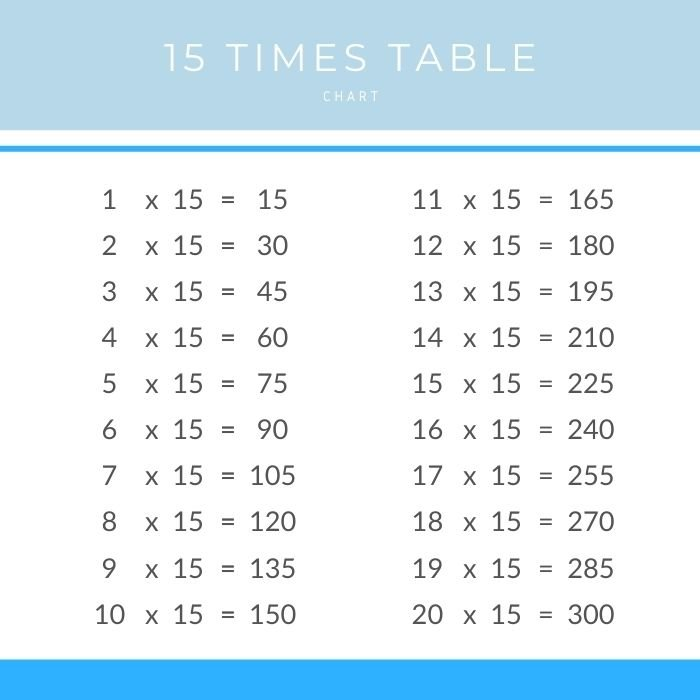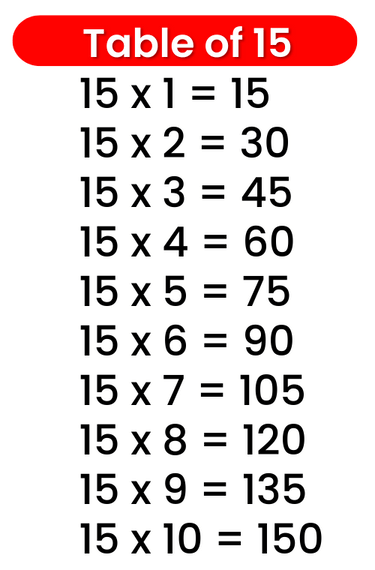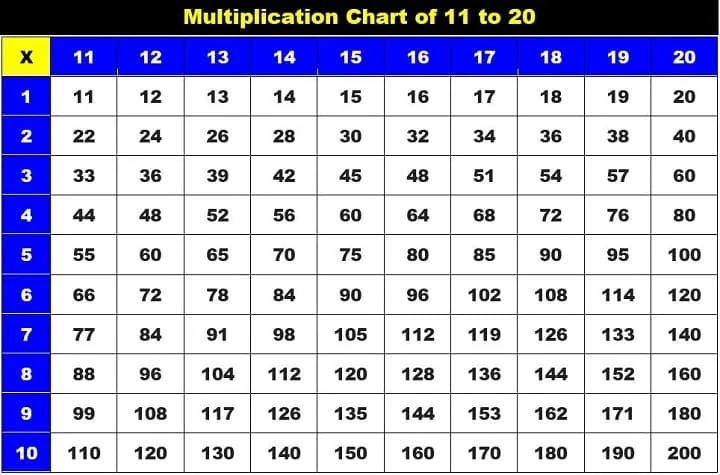# Times Table of 15, 15 ka table

## Times Table of 15, 15 ka table

Maths Multiplication Table of 15, Times Table of 15, 15 ka table, 15 ki Table Chart

15 ×‌ 1 = 15
15 ×‌ 2 = 30
15 ×‌ 3 = 45
15 ×‌ 4 = 60
15 ×‌ 5 = 75
15 ×‌ 6 = 90
15 ×‌ 7 = 105
15 ×‌ 8 = 120
15 ×‌ 9 = 135
15 ×‌ 10 = 150

## 15 ka table### Multiplication Tables of twelve upto 20### Times Table of 15 chart